# dielectric

Dielectric material for use as substrate

## Syntax

``d = dielectric(material)``
``d = dielectric(Name,Value)``

## Description

example

````d = dielectric(material)` returns dielectric materials for use as a substrate in antenna elements. ```

example

````d = dielectric(Name,Value)` returns dielectric materials, based on the properties specified by one or more `Name,Value` pair arguments.```

## Examples

collapse all

Use a Teflon dielectric material as a substrate for a PIFA antenna. View the antenna.

`d = dielectric('Teflon')`
```d = dielectric with properties: Name: 'Teflon' EpsilonR: 2.1000 LossTangent: 2.0000e-04 Thickness: 0.0060 For more materials see catalog ```
`p = pifa('Height',0.0060,'Substrate',d)`
```p = pifa with properties: Length: 0.0300 Width: 0.0200 Height: 0.0060 Substrate: [1x1 dielectric] GroundPlaneLength: 0.0360 GroundPlaneWidth: 0.0360 PatchCenterOffset: [0 0] ShortPinWidth: 0.0200 FeedOffset: [-0.0020 0] Conductor: [1x1 metal] Tilt: 0 TiltAxis: [1 0 0] Load: [1x1 lumpedElement] ```
`show(p)`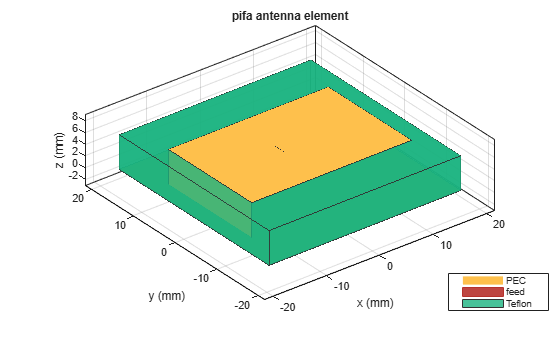Create a patch microstrip antenna using a substrate with a relative permittivity of 2.70, a loss tangent of 0.002 and a thickness of 0.0008 m. View the antenna.

```t = dielectric('Name','Taconic_TLC','EpsilonR',2.70,'LossTangent',0.002,... 'Thickness',0.0008); p = patchMicrostrip('Height',0.0008,'Substrate',t)```
```p = patchMicrostrip with properties: Length: 0.0750 Width: 0.0375 Height: 8.0000e-04 Substrate: [1x1 dielectric] GroundPlaneLength: 0.1500 GroundPlaneWidth: 0.0750 PatchCenterOffset: [0 0] FeedOffset: [-0.0187 0] Conductor: [1x1 metal] Tilt: 0 TiltAxis: [1 0 0] Load: [1x1 lumpedElement] ```
`show(p)`Create a microstrip patch antenna.

`p = patchMicrostrip;`

`For properties of air and teflon dielectrics use Dielectric Catalog.`

`openDielectricCatalog`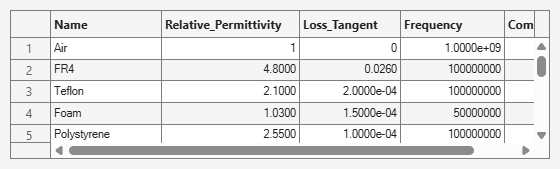Use Teflon as a dielectric substrate. There is an air gap between the patch groundplane and the dielectric.

```sub = dielectric('Name',{'Air','Teflon'},'EpsilonR',[1 2.1],... 'Thickness',[.002 .004],'LossTangent',[0 2e-04]);```

Add the substrate to the patch antenna.

```p.Substrate = sub; figure show(p)```Create a microstrip patch antenna.

`p = patchMicrostrip;`

For dielectric properties, use the Dielectric Catalog.

`openDielectricCatalog`Use FR4, Teflon and Foam as the three layers of the substrate.

```sub = dielectric('Name',{'FR4','Teflon','Foam'},'EpsilonR',... [4.80 2.10 1.03],'Thickness',[0.002 0.004 0.001],... 'LossTangent',[0.0260 2e-04 1.5e-04]);```

Add the three layer substrate to the patch antenna.

```p.Substrate = sub; figure show(p)```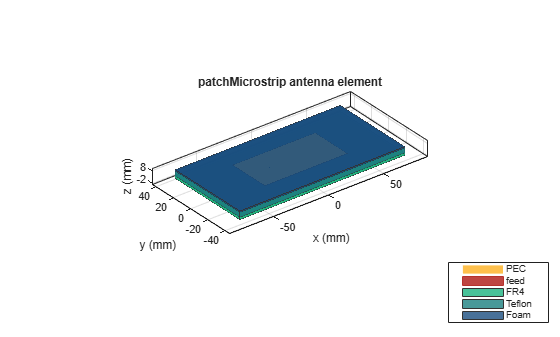Plot the radiation pattern of the antenna.

```figure pattern(p,1.67e9)```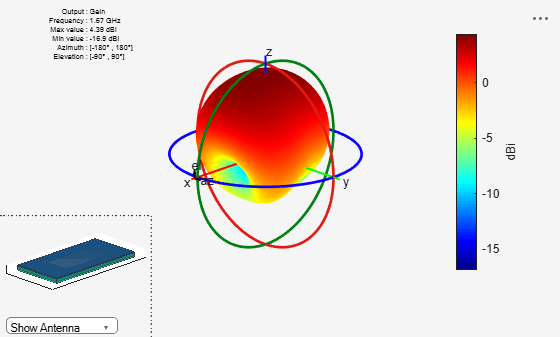Design a dipole antenna backed by a dielectric substrate and an infinite reflector.

Create a dipole antenna of length, 0.15 m, and width, 0.015 m.

`d = dipole('Length',0.15,'Width',0.015, 'Tilt',90,'TiltAxis',[0 1 0]);`

Create a reflector using the dipole antenna as an exciter and the dielectric, `teflon` as the substrate.

`t = dielectric('Teflon')`
```t = dielectric with properties: Name: 'Teflon' EpsilonR: 2.1000 LossTangent: 2.0000e-04 Thickness: 0.0060 For more materials see catalog ```
`rf = reflector('Exciter',d,'Spacing',7.5e-3,'Substrate',t);`

Set the groundplane length of the reflector to `inf`. View the structure.

```rf.GroundPlaneLength = inf; show(rf)```Calculate the radiation pattern of the antenna at 70 MHz.

`pattern(rf,70e6)`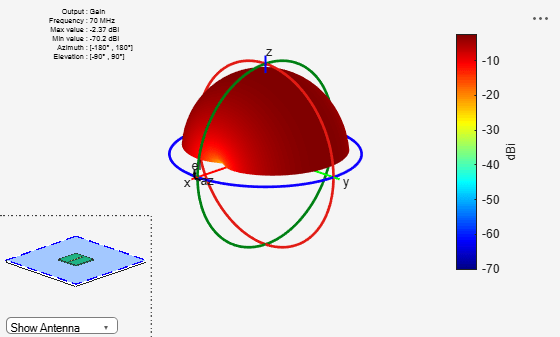Compare the gain values of a dipole antenna in free space and dipole antenna on a substrate.

Design a dipole antenna at a frequency of 1 GHz.

```d = design(dipole,1e9); l_by_w = d.Length/d.Width; d.Tilt = 90; d.TiltAxis = [0 1 0];```

Plot the radiation pattern of the dipole in free space at 1 GHz.

```figure pattern(d,1e9);```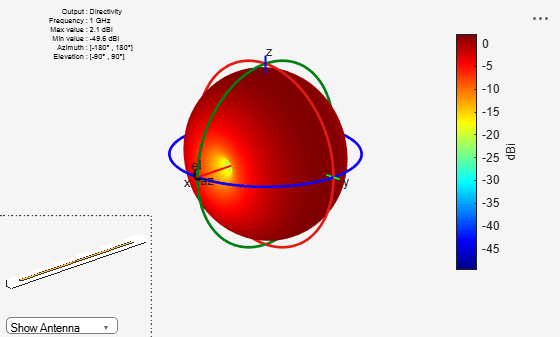Use FR4 as the dielectric substrate.

`t = dielectric('FR4')`
```t = dielectric with properties: Name: 'FR4' EpsilonR: 4.8000 LossTangent: 0.0260 Thickness: 0.0060 For more materials see catalog ```
```eps_r = t.EpsilonR; lambda_0 = physconst('lightspeed')/1e9; lambda_d = lambda_0/sqrt(eps_r);```

Adjust the length of the dipole based on the wavelength.

```d.Length = lambda_d/2; d.Width = d.Length/l_by_w;```

Design a reflector at 1 GHz with the dipole as the exciter and FR4 as the substrate.

```rf = reflector('Exciter',d,'Spacing',7.5e-3,'Substrate',t); rf.GroundPlaneLength = lambda_d; rf.GroundPlaneWidth = lambda_d/4; figure show(rf)```Remove the groundplane for plotting the gain of the dipole on the substrate.

```rf.GroundPlaneLength = 0; show(rf)```Plot the radiation pattern of the dipole on the substrate at 1 GHz.

```figure pattern(rf,1e9);```Compare the gain values.

• Gain of the dipole in free space = 2.11 dBi

• Gain of the dipole on substrate = 1.93 dBi

## Input Arguments

collapse all

Material from the dielectric catalog, specified as one of the values from the `DielectricCatalog`.

Example: `'FR4'`

Data Types: `char`

### Name-Value Arguments

Specify optional comma-separated pairs of `Name,Value` arguments. `Name` is the argument name and `Value` is the corresponding value. `Name` must appear inside quotes. You can specify several name and value pair arguments in any order as `Name1,Value1,...,NameN,ValueN`.

Example: `'Name'`,`'Air'`

Name of the dielectric material you want to specify in the output, specified as the comma-separated pair consisting of `'Name'` and a character vector.

Example: `'Name'`,`'Taconic_TLC'`

Data Types: `char`

Relative permittivity of the dielectric material, specified as the comma-separated pair consisting of `'EpsilonR'` and vector.

Example: `'EpsilonR'`,`4.8000`

Data Types: `double`

Loss in the dielectric material, specified as the comma-separated pair consisting of `'LossTangent'` and vector.

Example: `'LossTangent'`,`0.0260`

Data Types: `double`

Note

In Antenna Toolbox™, the upper limit to loss tangent value is 0.03.

Thickness of the dielectric material along default z-axis, specified as the comma-separated pair consisting of `'Thickness'` and vector in meters. This property applies only when you call the function with no output arguments.

Example: `'Thickness'`, `0.05`

Data Types: `double`

## Output Arguments

collapse all

Dielectric material, returned as an object. You can use the dielectric material object to add dielectric material to an antenna.